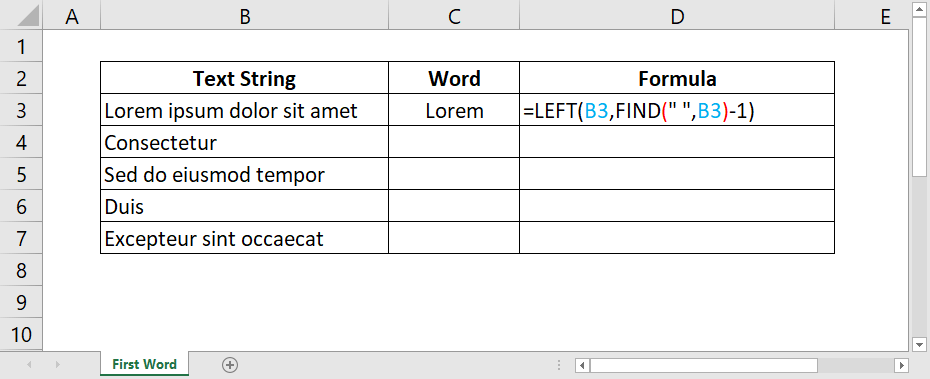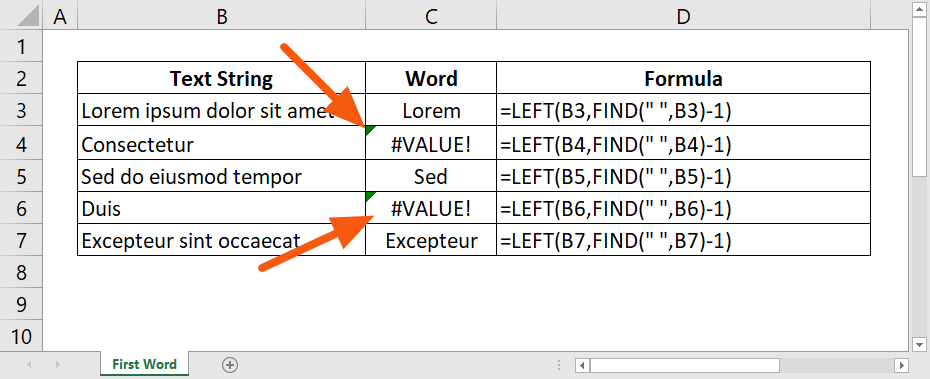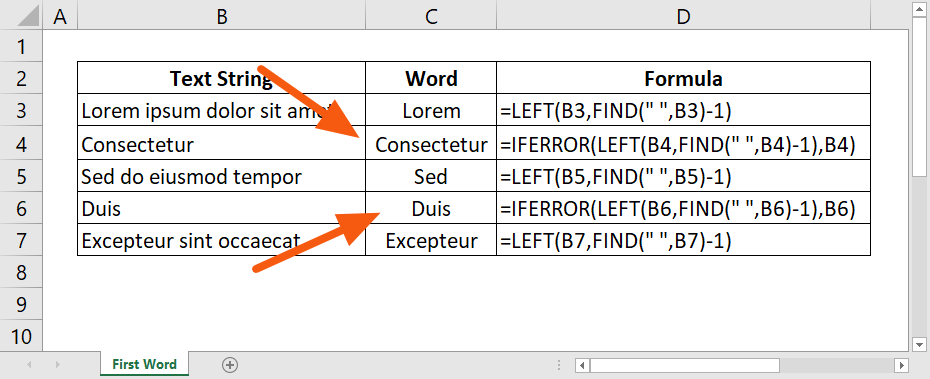# How to Grab The First Word of a Text String in Excel

Written by co-founder Kasper Langmann, Microsoft Office Specialist.

Is it possible to grab the first word of a text string in Excel?

Yes, it definitely is!

However, there’s no button that will do that. You would need to use a combination of functions.

In this article, we’ll show you how to get the first word of a text string in Excel.

Let’s get started!

*This tutorial is for Excel 2019/Microsoft 365 (for Windows). Got a different version? No problem, you can still follow the exact same steps.

## How to get the first word of a string

To make it work, you need to use two functions, ‘LEFT’ and ‘FIND’.

The ‘LEFT’ function returns the characters from the left side of a string.

The syntax of the ‘LEFT’ function

`=LEFT (text, [num_chars])`

The ‘text’ parameter is where the function will extract the characters from.

The ‘num_chars’ parameter is optional. It refers to the number of characters to extract from the left side of the string.

The ‘FIND’ function, on the other hand, returns the position of a text string inside another, as a number.

The syntax of the ‘FIND’ function

`=FIND (find_text, within_text, [start_num])`

Parameters:

• ‘find_text’ = the text string to find
• ‘within_text’ = the location or text string to search from
• ‘start_num’ = optional; the starting position in the text to search from

To grab the first word of a string, all you need to do is nest the ‘FIND’ function inside the ‘LEFT’ function in such manner:

`=LEFT(text, FIND(" ", within_text) -1)`

This works by having the ‘FIND’ function search for the position of the first occurrence of a space character (“ “) in the text.

The result of the ‘FIND’ function minus 1 will then be fed into the ‘LEFT’ function as it extracts the characters starting the left side of the text or position -1.

Here’s how it looks in action:Easy, right?

There’s just one problem with this:

It will return a #VALUE! error if the text string has one word only.

Like this:## Get your FREE exercise file

Before you start:

Throughout this guide, you need a data set to practice.

## How to avoid errors

To avoid an error if there’s only one word in the string, you have to wrap the formula with the ‘IFERROR’ function.

The idea behind this function is simple — to return a custom result if the formula generates an error.

The syntax of the ‘IFERROR’ function

`=IFERROR (value, value_if_error)`

Parameters:

• ‘value’ = the formula to check for an error
• ‘value_if_error’ = the custom value to return if there’s an error found

Nesting the formula we used earlier with ‘IFERROR’ will result in this:

`=IFERROR(LEFT(text, FIND(" ", within_text) -1), value_if_error)`

Here it is in action:Nice and easy! 😉

## Wrapping things up…

As you can see, it’s not really that hard to grab the first word of a text string in Excel. All you have to do is combine the ‘FIND’ and ‘LEFT’ functions.

However, using such a formula with a text string with only one word will return an error. To combat this, you have to use the ‘IFERROR’ function to wrap the formula and return a custom value when there’s an error found.

If you find yourself having a hard time wrapping your head around this, feel free to download the exercise file included in this tutorial. Then, simply copy the formula outlined here and study it out. 😊